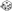General Use Thread - Page 28 - Myth-Weavers

 Notices The winners of the first Storycrafting contest have been announced! Congratulations to our grand prize winner, Underleaf, and the runners up!

# General Use Thread

Great Wyrm

Dice Roll: 1d20+12z 1d20+12z
d20 Results: 7 (Total = 19)
d20 Results: 5 (Total = 17)Barlo's Knowledge Local on Giants (19,17)
Dice Roll: 1d20+9z
d20 Results: 18 (Total = 27)Barlo's Knowledge Nature on Rat (27)

Great Wyrm

Dice Roll: 1d20+13z 1d20+8z
d20 Results: 4 (Total = 17)
d20 Results: 3 (Total = 11)Full Attack on S2 (17,11)
Dice Roll: 1d8+9z 1d8+9z
d8 Results: 7 (Total = 16)
d8 Results: 1 (Total = 10)Damage (16,10)

light mace is considered a light weapon...so attack bonus is +10, plus there is insightful strike on the dmg. but insightful doesn't matter since undead are immune...

Dice Roll: 1d20+10z 1d20+5z
d20 Results: 19 (Total = 29)
d20 Results: 16 (Total = 21)Full Round attack (29,21)
Dice Roll: 1d6+2z 1d6+2z
d6 Results: 3 (Total = 5)
d6 Results: 1 (Total = 3)dmg (5,3)

Great Wyrm

Hellsing attack S2 with PBS and +2 favored enemy undead -

Dice Roll: 1d20+12z
d20 Results: 13 (Total = 25)

Damage -

Dice Roll: 1d8+6z
d8 Results: 5 (Total = 11)

Skirmish damage -

Dice Roll: 2d6z
d6 Results: 2, 3 (Total = 5)

Great Wyrm

Dice Roll: 1d20+18z 1d20+18z 1d20+18z 1d20+18z 1d20+18z
d20 Results: 19 (Total = 37)
d20 Results: 8 (Total = 26)
d20 Results: 13 (Total = 31)
d20 Results: 1 (Total = 19)
d20 Results: 5 (Total = 23)Melisande Fort Saves (37,26,31,19,23)
Dice Roll: 1d20+8z 1d20+8z 1d20+8z 1d20+8z 1d20+8z 1d20+8z 1d20+8z 1d20+8z 1d20+8z 1d20+8z
d20 Results: 6 (Total = 14)
d20 Results: 7 (Total = 15)
d20 Results: 14 (Total = 22)
d20 Results: 9 (Total = 17)
d20 Results: 6 (Total = 14)
d20 Results: 12 (Total = 20)
d20 Results: 6 (Total = 14)
d20 Results: 13 (Total = 21)
d20 Results: 1 (Total = 9)
d20 Results: 8 (Total = 16)Horse Fort Saves (14,15,22,17,14,20,14,21,9,16)

Great Wyrm

Dice Roll: 1d20+9z
d20 Results: 4 (Total = 13)Ride Check to negate Attack on Justiel (13)

Great Wyrm

At smaller giant
Dice Roll: 1d20+12z 1d6+4z
d20 Results: 1 (Total = 13)
d6 Results: 2 (Total = 6)1st Arrow (13,6)
Dice Roll: 1d20+12z 1d6+4z
d20 Results: 7 (Total = 19)
d6 Results: 2 (Total = 6)2nd Arrow (19,6)
Dice Roll: 1d20+7z 1d6+4z
d20 Results: 15 (Total = 22)
d6 Results: 2 (Total = 6)3rd Arrow (22,6)

Great Wyrm

Dice Roll: 1d20+13z 3d8+18z
d20 Results: 20 (Total = 33)
d8 Results: 3, 4, 5 (Total = 30)Lance (33,30)
Dice Roll: 1d20+8z 1d6+3z
d20 Results: 16 (Total = 24)
d6 Results: 6 (Total = 9)Justiel Hoof (24,9)
Dice Roll: 1d20+9z
d20 Results: 19 (Total = 28)Ride Check to negate Attack (28)

Dice Roll: 1d20+13z 2d8+12z
d20 Results: 11 (Total = 24)
d8 Results: 8, 4 (Total = 24)Crit Confirm Lance (24,24)

Great Wyrm

Justiel Full Attack on H1
Dice Roll: 1d20+5z 1d4+3z
d20 Results: 19 (Total = 24)
d4 Results: 2 (Total = 5)Bite (24,5)
Dice Roll: 1d20+6z 1d6+3z
d20 Results: 10 (Total = 16)
d6 Results: 3 (Total = 6)Hoof 1 (16,6)
Dice Roll: 1d20+6z 1d6+3z
d20 Results: 20 (Total = 26)
d6 Results: 1 (Total = 4)Hoof 2 (26,4)

Dice Roll: 1d20+9z
d20 Results: 10 (Total = 19)Gaius Ride Check to avoid attack on Justiel (19)

Dice Roll: 1d20+10z 1d8+6z
d20 Results: 16 (Total = 26)
d8 Results: 7 (Total = 13)Gaius AoO on H3 (26,13)
Dice Roll: 1d20+10z 1d8+4z
d20 Results: 13 (Total = 23)
d8 Results: 2 (Total = 6)Gaius Longsword on H1 (23,6)

Great Wyrm

Dice Roll: 1d20+16z
d20 Results: 1 (Total = 17)Barlo's Acrobatics (17)